# 44 Copy and Move Formulas

 <  Day Day Up  >

You can copy, move, and paste one cell into another using standard copy-and-paste tools such as the Windows Clipboard. When you copy formulas that contain cell addresses, Calc updates the cell references so they become relative references . For example, suppose that you enter this formula in cell A1 :

` `

`  =A2 + A3  `

Before You Begin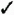43 About Calc Formulas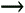49 Create a Range

This formula contains two cell references. The references are relative because the references A2 and A3 change if you copy the formula elsewhere. If you copy the formula to cell B5 , for example, B5 holds this:

` `

` =B6 + B7 `

KEY TERM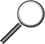Relative reference ” A cell that is referenced in relation to the current cell.

The original relative references update to reflect the formula's copied location. Of course, A1 still holds its original contents, but the copied cell at B5 holds the same formula referencing B5 rather than A1 .

KEY TERMAbsolute reference ” A cell reference that does not change if you copy the formula elsewhere.

A dollar sign (\$) always precedes an absolute reference . The reference \$B\$5 is an absolute reference. If you want to sum two columns of data ( A1 with B1, A2 with B2 , and so on) and then multiply each sum by some constant number, for example, the constant number can be a cell referred to as an absolute reference . That formula might resemble this:

` `

` =(A1 + B1) * \$J `

In this case, \$J\$1 is an absolute reference, but A1 and B1 are relative. If you copy the formula down one row, the formula changes to this:

` `

` =(A2 + B2) * \$J `

Notice that the first two cells changed because when you originally entered them, they were relative cell references. You told Calc, by placing dollar signs in front of the absolute cell reference's row and column references, not to change that reference when you copy the formula elsewhere.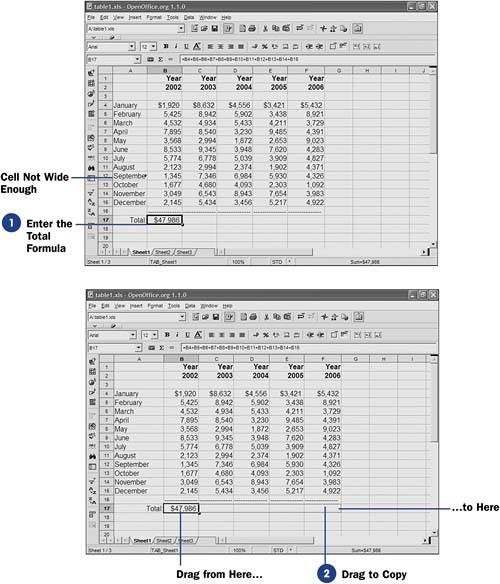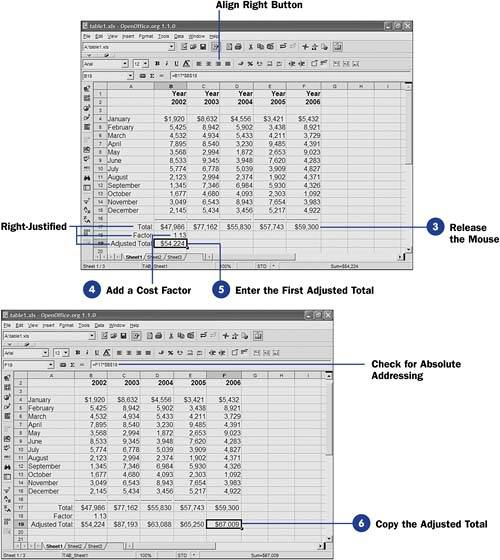\$B5 is a partial absolute cell reference. If you copy a formula with \$B5 inside the computation, the \$B keeps the B column intact, but the fifth row updates to the row location of the target cell. For example, if you type the formula

` `

`  =2 * \$B5  `

TIP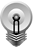Most of the time, you'll use relative referencing. If you insert or delete rows, columns, or cells, your formulas remain accurate because the cells that they reference change as your worksheet changes.

in cell A1 and then copy the formula to cell F6 , cell F6 holds this formula:

` `

` =2 * \$B10 `

You copied the formula to a cell five rows and five columns over in the worksheet. Calc did not update the column name , B , because you told Calc to keep that column name absolute. (It is always B no matter where you copy the formula.) Calc added five to the row number, however, because the row number is relative and open to change whenever you copy the formula.

TIPThere are several better ways to total a column. The most tedious but easiest to understand is this step's way. One better way would be to type this formula: =Sum(B4:B15 ) . 51 About Calc Functions explores Sum() and other Calc functions.

1. Enter the Total Formula

For this sheet, assume you want a formula for each past year and projected year into the future. You would type the formula to total the first year in cell B17 . One formula that would total this year would be

` `

` =B4 + B5 + B6 + B7 + B8 + B9 + B10 + B11 + B12 + B13 + B14 + B15 `

Notice that cell A12 does not display all of the month of September's name. The column is not wide enough to display the full month. Calc warns you that this cell's contents aren't fully displayed with a small triangle along the right side of the cell. You can widen cells like this that you find are too narrow by dragging the dividing line between the name of column A and column B to the right to give every month name enough room to display properly.

A quick way to resize a column to ensure that it is wide enough for all its data is to double-click the column divider between the column you want to widen and the one on its right. Calc automatically resizes the column width to hold the widest value in the column.

2. Drag to Copy

Once you type a formula in cell B17 , you can copy that cell to the Windows Clipboard with Ctrl+C and then paste the cell into C17 , then D17 , then E17 , and finally F17 . Calc offers an easier way though. Drag the lower-right corner of cell B17 , where you'll see a small square, to the right, and Calc highlights each empty cell along the way as you copy.

NOTE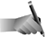When you drag your mouse across multiple cells, you are indicating a range that you want to work with.

NOTELook at the Input line for cell F17 . You'll see that Calc copied the formula from B17 using relative addressing. It wouldn't make sense to put the total for column B in cell F17 . When Calc saw that you wanted to copy the formula, and because the formula contains relative cell references, Calc copied the formula as though it referred to cells relative to B17 .
3. Release the Mouse

Once you release your mouse button over cell F17 , you will see that Calc totals all five columns for you where you copied the total formula.

To demonstrate absolute cell addressing, add a cost factor of 1.13 below Year 2002 's total. You then can multiply the total to create an adjusted total in cell B19 . If you also want to multiply the remaining totals by the adjustment factor, you cannot use relative addressing for the cost factor. In other words, if you multiply cell B17 by cell B18 and store the result in B19 with the formula =B17*B18 , and then copy that formula to cell C19 , cell C19 would hold this formula: =C17*C18 . However, C18 is blank! So Calc would multiply C17 by zero, which is not the correct adjustment factor.

NOTEThe figure's cells are formatted to display dollar signs in some places and not in others. 59 Format Cells explains how to format cells the way you want them to look. The labels for Factor and Adjusted Total are right-justified (with the Align Right button); how-ever, they first enter their respective cells left-justified because they contain text.
5. Enter the First Adjusted Total

To enter the correct adjusted total in cell B19 , you would type =B17 * \$B\$18 .

By using absolute addressing in cell B18 (that is, \$B\$18 ), when you copy it to the remaining years , all the cells you copy to will also use B18 instead of a different cell for the factor.

To copy the adjusted total to the other years, you can use Ctrl+C and then paste with Ctrl+V into each year's adjusted total cell, but it's simpler just to drag the small square in the first cell's lower-right corner (the mouse pointer changes to a plus sign when you point to this square) across through the cells that are to receive the copied formula.

TIPYou don't need to leave spaces between operators such as multiplication (*) in formulas. Doing so makes them easier to read and to check for errors, however.
When you release your mouse after making such a copy with one or more absolute cell addresses in the range, the absolute address remains the same and the relative addresses inside the cells change. This sounds less obvious than it is. In other words, when you copy the formula =B17 * \$B\$18 to cell C19 , cell C19 gets this formula: =C17 * \$B\$18 . Cell D19 gets =D17 * \$B\$18 , and so on.

NOTEYou can make only the row or only the column of a cell address absolute. In the cell reference M\$15 , the column named M is relative and will change if you copy a cell that contains this reference elsewhere, but the absolute row number, \$15 , will not change.

 <  Day Day Up  >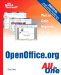Sams Teach Yourself OpenOffice.org All In One
ISBN: 0672326183
EAN: 2147483647
Year: 2003
Pages: 205
Authors: Greg Perry

Similar book on Amazon